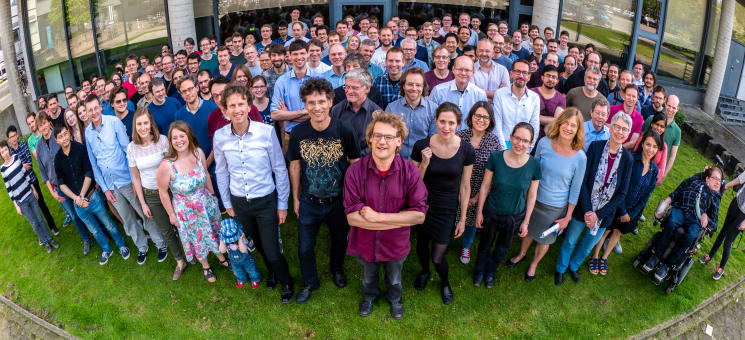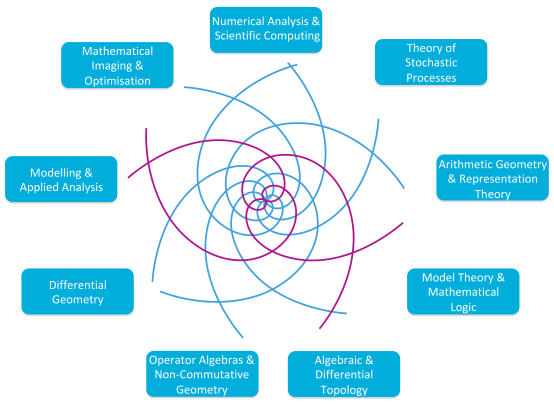© WWU

We aim to further develop mathematics in Münster into a research centre with high international visibility. We will tackle fundamentally important mathematical problems, viewing mathematics as an organic whole with countless interactions.

In order to reach our goals, we will follow three key principles: connecting mathematical fields, supporting early career researchers, and improving equal opportunity and compatibility of family and career.© MM

Mathematics Münster provides an umbrella integrating the four mathematical institutes of the WWU (Mathematical Institute, Institute for Analysis and Numerics, Institute for Mathematical Stochastics and the Institute for Mathematical Logic and Foundations of Mathematics) in order to foster collaboration between the 16 mathematical fields and scientific exchange. Almost 50 researchers collaborate on important mathematical problems in research areas Arithmetic and Groups (A), Spaces and Operators (B), and Models and Approximations (C). Our research is unified by three major threads: the emphasis on the underlying structures of a given problem, the geometric viewpoint and the study of the relevant dynamics of group or semigroup actions.